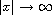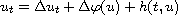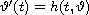Electron. J. Diff. Equ., Vol. 2012 (2012), No. 141, pp. 1-12.

### Behavior of the maximal solution of the Cauchy problem for some nonlinear pseudoparabolic equation asTatiana Kavitova

Abstract:
We prove a comparison principle for solutions of the Cauchy problem of the nonlinear pseudoparabolic equationwith nonnegative bounded initial data. We show stabilization of a maximal solution to a maximal solution of the Cauchy problem for the corresponding ordinary differential equationasunder certain conditions on an initial datum.

Submitted March 22, 2012. Published August 20, 2012.
Math Subject Classifications: 35B40, 35B51, 35K70.
Key Words: Pseudoparabolic equation; comparison principle; stabilization.

Show me the PDF file (272 KB), TEX file, and other files for this article.Tatiana Kavitova Department of Mathematics, Vitebsk State University Moskovskii pr. 33, 210038 Vitebsk, Belarus email: KavitovaTV@tut.by